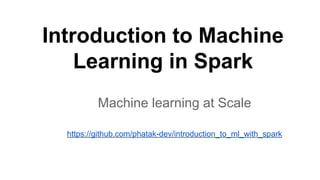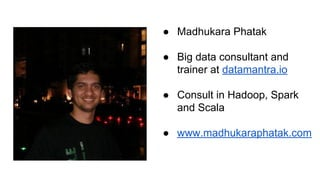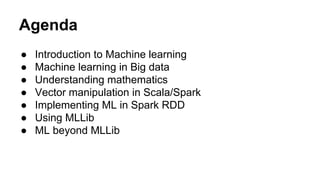Seu SlideShare está sendo baixado. ×

# Introduction to Machine Learning with Spark

Anúncio
Anúncio
Anúncio
Anúncio
Anúncio
Anúncio
Anúncio
Anúncio
Anúncio
Anúncio
AnúncioCarregando em…3
×

1 de 52 Anúncio

# Introduction to Machine Learning with Spark

Talk about implementing machine learning in Apache Spark.Presented at Bangalore Spark meetup group at Bangalore by Madhukara Phatak on 05/09/2015.

Talk about implementing machine learning in Apache Spark.Presented at Bangalore Spark meetup group at Bangalore by Madhukara Phatak on 05/09/2015.

Anúncio
Anúncio

### Introduction to Machine Learning with Spark

1. 1. Introduction to Machine Learning in Spark Machine learning at Scale https://github.com/phatak-dev/introduction_to_ml_with_spark
2. 2. ● Madhukara Phatak ● Big data consultant and trainer at datamantra.io ● Consult in Hadoop, Spark and Scala ● www.madhukaraphatak.com
3. 3. Agenda ● Introduction to Machine learning ● Machine learning in Big data ● Understanding mathematics ● Vector manipulation in Scala/Spark ● Implementing ML in Spark RDD ● Using MLLib ● ML beyond MLLib
4. 4. 3 D’s of big data processing ● Data Scientist Models simple world view from chaotic complex real world data ● Data Engineer Implements simple model on complex toolset and data ● Data Artist Explains complex results in simple visualizations
5. 5. Introduction to Machine Learning
6. 6. What is machine learning? A computer program is said to learn from experience E with respect to some task T and some performance measure P, if it’s performance on T, as measured P, improves with experience E - Tom Mitchell (1998)
7. 7. How human learn? ● We repeat the same task (T) over and over again to gain experience (E) ● This doing over and over again same task is known as practice ● With practice and experience, we get better at that task ● Once we achieve some level,we have learnt ● Everything in life is learnt
8. 8. Learning example - Music instrument ● We practice each day the instrument with same song again and again ● We will be pretty bad to start with, but with practice we improve ● Our teacher will measure performance to understand did we done progress ● Once your teacher approves, you are a player
9. 9. Why it’s hard to learn? ● Practicing over and over same task is often boring and frustrating ● Without proper measurement, we may be stuck at same level without making much progress ● Making progress initially is pretty easy but it’s starts to get harder and harder ● Sometime natural talent also dicates cap on how best we can be
10. 10. How machine learn? ● You start with an assumption about solution for given problem ● Make machine go over data and verify the assumption ● Run the program over and over again on same data updating assumption on the way ● Measure improvement after each round, adjust accordingly ● Once you have no more assumption changing, stop running
11. 11. Brute force machine learning Problem : Find relation between x , y and z values , given data set x y z 1 2 5 2 3 6 2 4 7 What human approach to this?
12. 12. ML approach ● Let’s assume that x,y,z are connected as below z = c0 + c1x + c2y where c0, c1, c2 factors which we need to learn ● Let’s start with following values c0 = 0 c1 = 1 c2 = 1 ● Find out the error for each row ● Adjust the values for c0,c1,c2 from error ● Repeat
13. 13. ML history ● Sub branch of AI ● Impossible to model brain because it's not an ideal world ● Most of the time we don’t need all complexity of real world ex : ideal gas equation from thermodynamics ● Approximation of real world is more than enough to solve real problems
14. 14. ML and Data ● The quality of a model of ML is determined by ○ learning algorithm ○ Amount of data it has seen ● More the data, a simple algorithm can do better than sophisticated algorithm with less data ● So for long ML was focused coming up with better algorithms as amount of data was limited ● Then we landed on big data
15. 15. Machine learning in Big data
16. 16. ML and Big data ● Ability to learn on large corpus of data is a real boon for ML ● Even simplistic ML models shine when they are trained on huge amount of data ● With big data toolsets, a wide variety of ML application have started to emerge other than academic specifics one ● Big data democratising ML for general public
17. 17. ML vs Big data Machine Learning Big data Optimized for iterative computations Optimized for single pass computations Maintains state between stages Stateless CPU intensive Data intensive Vector/Matrix based or multiple rows/cols at a time Single column/ row at time
18. 18. Machine learning in Hadoop ● In Hadoop M/R each iteration of ML translates into single M/R job. ● Each of these jobs need to store data in HDFS which creates a lot of overhead ● Keeping state across jobs is not directly available in M/R ● Constant fight between quality of result vs performance
19. 19. Machine learning in Spark ● Spark is first general purpose big data processing engine build for ML from day one ● The initial design in Spark was driven by ML optimization ○ Caching - For running on data multiple times ○ Accumulator - To keep state across multiple iterations in memory ○ Good support for cpu intensive tasks with laziness ● One of the examples in Spark first version was of ML
20. 20. First ML Example in Spark
21. 21. Understanding Mathematics behind ML
22. 22. Major types of ML ● Supervised learning Training data contains both input vector and desired output. We also called it as labeled data Ex : Linear Regression, Logistic Regression ● Unsupervised learning Training data sets without labels. Ex : K-means clustering
23. 23. Process of Supervised Learning Training Set Learning Algorithm Hypothesis/model New data Result (Prediction/Classification)
24. 24. ML Terminology ● Training Set Set of data used to teach machine. In supervised learning both input vector and output will be available. ● Learning algorithm Algorithm which consumes the training set to infer relation between input vectors which optimises for known output labels ● Model Learnt function of input parameters
25. 25. Linear regression ● A learning algorithm to learn relation between dependent variable y with one or more explanatory variable x which are connected by linear relation. ● Given multiple dimensional data, the hypothesis for linear regression looks as below h(x) = c0 + c1x1 + c2x2 + …. ● x1, x2 are the explanatory variables and y is the dependent variable. h(x) is the hypothesis. ● Linear regression goal is to learn c0,c1,c2
26. 26. Linear Regression Example ● Price of the house is dependent on size of the house ● What will be price of the house if size of the house is 1200? Size of the house(sft) Price(in Rs) 1000 5 lakh 2000 15 lakh 800 4 lakh
27. 27. Linear regression for housing data ● Price of the house is dependent on size of the house ● So in linear regression y -> price of the house x -> size of the house ● Then model we have to learn is Price_of_the_house = c0+ c1* size_of_the_house ● We assume relationship is linear
28. 28. Curve fitting
29. 29. Choosing values ● Our model is h(x) = c0 + c1x1 + c2x2 + …. ● How to choose c0, c1, c2? ● We want to choose c0,c1,c2 such way which gives us y values which are close to the one in training set ● But how we know they are close to y? ● Cost function helps to find out they are close or not.
30. 30. Cost function ● Cost function for linear regression is J(c) = (h(x) - y)^2 / 2m - know as squared error ● J(c) is a function of c0,c1,c2 because cost changes depending upon the different c1, c2 ● m is number of rows in training data ● Goal of learning algorithm is to learn a model which minimizes this error ● How to minimize J(c)?
31. 31. Curve of cost function
32. 32. Minimising cost function ● Start with some random value of c0, c1 ● Calculate the cost ● Keep on changing c0, c1 till you find the minimum cost ● Gradient descent in one of the algorithm to find minimum any mathematical function ● Also known as convex optimizer ● The name gradient comes from, use of gradient of the function to decide which way to walk
33. 33. Gradient descent ● The way to update c values in gradient descent c(j) = c(j) - alpha * derivative( J(c)) ● alpha is known as learning rate ● For linear regression ○ cost function - J(c) = (h(x) - y)^2 / 2m ○ Derivative is = ■ c0 - (h(x) - y) / m ■ c1,c2 .. - ((h(x) - y) * x ) / m
35. 35. Linear regression algorithm ● Start with a training set with x1,x2, x3 .. and y ● Start with parameters c0,c1,c3 with random values ● Start with a learning rate alpha ● Then repeat the following update c0 = c0 - alpha * h(x) - y c1 = c1 - alpha * (h(x) - y) * x ● Repeat this process till it converges
36. 36. Vectors in ML ● All information in machine learning is represented using vectors of numbers which represent features ● Collection of vectors are represented as matrices ● All the calculation in machine learning are expressed using vector manipulation ● So Understanding vector manipulation is very important ● We will understand how Scala and Spark represents the vector
37. 37. Vector manipulation in Scala/Spark
38. 38. Breeze - Vector library for Scala ● Breeze is library for numerical processing for scala ● Option to use fortran based native numeric library using netlib-java ● Spark uses breeze internally for vector manipulation in MLLib library ● Many data structures of MLLib are modeled around breeze data structures ● https://github.com/scalanlp/breeze
39. 39. Representing data in breeze vector ● Two types of vectors ○ Dense vectors ○ Sparse vectors ● All vectors in breeze are column vectors. Take transpose for row vector ● Normally row vectors are used to represent the data and column vectors for representing weights or coefficients of learning algorithm ● VectorExample.scala
40. 40. Vector/Matrix manipulation ● Multiplying vector from a constant ○ Multiply using netlib ○ Multiply using constant vector elementwise MultiplyVectorByConstant.scala ● Multiplying matrix from a constant ○ value ○ vector MultiplyMatrixByConstant.scala
41. 41. Dot product ● Dot product of two vectors A = [ a1, a2 , a3 ..] and B= [b1,b2,b3 .. ] is A.B = sum ( a1*b1 + a2*b2 + a3*b3) ● Two ways to implement in breeze ○ A.dot(B) ○ Transpose(A) * B) ● DotProductExample.scala
42. 42. In-place computations ● Creating a breeze vector/matrix is a costly operations ● So if each transformation in our computation creates new vector, it may hurt our performance ● We can use in place operations, which can update existing vectors rather than creating new vector ● When we do many vector manipulations, in-place is preferred for better performance ● Ex : InPlaceExample.scala
43. 43. Representing data as RDD[Vector] ● In Spark ML, each row is represented using vectors ● Representing row in vector allows us to easily manipulate them using earlier discussed vector manipulations ● We broadcast vector for efficiency ● We can manipulate partition at a time using represent them as the matrices ● Manipulating as partition can give good performance ● RDDVectorExample.scala
44. 44. Implementing Linear Regression in Spark
45. 45. Implementing LR in Spark ● Represent that data as DataPoint which has ○ x - feature vector ○ y - value to be predicted ● Use accumulator to keep track of the cost ● Use reduce to aggregate gradient across multiple rows ● Uses gradient descent to work on this ● Ex : LinearRegressionExample.scala
46. 46. Stochastic gradient descent ● Use mini batch to do rather complete dataset ● Using sampling we can achieve this ● Mini batches help to speed up the gradient descent in multiple level ● By default batch size is 1.0 ● Ex : LRWithSGD.scala
47. 47. Using MLLib
48. 48. MLLib ● Machine learning library shipped with standard distribution of Spark ● Supports popular machine learning algorithms like Linear regression, Logistic regression, decision trees etc out of the box ● Every release new algorithms are added ● Supports multiple optimization techniques SGD, LBFGS
49. 49. Linear Regression in MLLib ● org.apache.spark.mllib.linalg.DenseVector wraps breeze vector for MLLib library ● Use LabeledPoint to represent the data of a given row ● Built in support for Linear regression with SGD ● Ex :LinearRegressionInMLLib.scala
50. 50. LR for housing data ● We are going to predict housing price using house size ● Size and price are in different scale ● Need to scale both of them to same scale ● We use StandardScaler to scale RDD[Vector] ● Scaled Data will be used for LinearRegression ● Ex : LRForHousingData.scala
51. 51. Machine learning beyond MLLib ● MLLib Pipelines API ● MLLib feature framework ● Sparkling water http://h2o.ai/product/sparkling-water/ ● SparkR ● http://prediction.io/
52. 52. References ● https://www.coursera.org/learn/machine-learning ● https://github.com/zinniasystems/spark-ml-class ● https://github.com/scalanlp/breeze